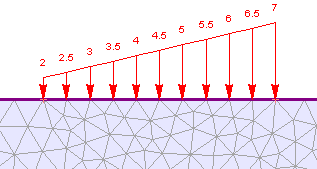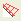## Search Results

The Add Triangular Load option allows you to define a distributed load which varies linearly between two points along a boundary. The load can be triangular (zero at one end) or trapezoidal (different non-zero values at each end).To apply a triangular or trapezoidal distributed load:

1. Select Add Triangular Loadfrom the toolbar or the Distributed Loads sub-menu of the Loading menu.
2. You will see the Add Distributed Load dialog. In this dialog:
• select the load orientation and enter the load magnitudes (two magnitudes are required, for the start and end points of the load)
• in a multi-stage model, distributed loads can be staged by selecting the Stage Load check box, and entering a Factor for the load magnitudes at each stage. If you do NOT stage the load on a multi-stage model, then the load will be applied with the same magnitude at all stages.
3. See below for details about the load magnitude, orientation and staging.

4. select [OK] in the dialog. Now you must graphically select the start and end points of the load, as described below.
5. NOTE: the start and end points must be on vertices of a boundary. If a vertex does not exist at the point where you wish to start or end the load, then you will have to reset the mesh, add the required vertex or vertices to the boundary, and re-mesh, before you can add the load.

6. Use the mouse to click on the starting vertex of the load.
7. Now move the mouse along the desired boundary. As you track the mouse along the boundary, a dotted line will highlight the boundary, and the load will be drawn on the screen. NOTE:
• a triangular load can span any number of segments on the same boundary.
• a triangular load can only be added along a single boundary (e.g. you cannot begin a triangular load on an excavation boundary, and then switch to a stage boundary). If you need to define a triangular load across different connecting boundaries, then the load will have to be split up into appropriate individual loads, and each one added separately.
• Flip Angle - if the load is pointing in the wrong direction (i.e. opposite from the intended direction), then you can use the Flip Angle option to reverse the load direction. You can right-click the mouse and select Flip Angle from the popup menu, or type f in the prompt line followed by Enter.
8. When you have reached the desired endpoint vertex, click the mouse and the load will be added to the model. NOTE: you must click the mouse when the cross-hair icon is ON a vertex of the boundary, in order to add the load. If you click the mouse at an intermediate location along the boundary (not a vertex location), then nothing will happen (you will remain in the add load mode).

## Load Magnitude, Orientation and Staging

For details about defining the load magnitude, orientation and staging, see the Add Uniform Load topic, as the same information applies to both Uniform and Triangular distributed loads.

NOTE: for a Triangular load, if you are staging the load magnitude, you may define different stage factors at each end of the load by selecting the Different stage factors on each end check box in the Stage Factors dialog.